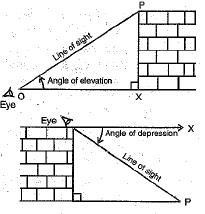Courses

# Important definitions and formulas - Some Application of Trigonometry Class 10 Notes | EduRev

## Class 10 : Important definitions and formulas - Some Application of Trigonometry Class 10 Notes | EduRev

The document Important definitions and formulas - Some Application of Trigonometry Class 10 Notes | EduRev is a part of the Class 10 Course Mathematics (Maths) Class 10.
All you need of Class 10 at this link: Class 10

⇒ Line of sight: The line drawn from the eye of an observer to a point in the object where the person is viewing is called the line of sight.
⇒ Angle of elevation: The angle formed by a line of sight OP with the horizontal line OX when the object is above the horizontal line is called the angle of elevation.
⇒ Angle of depression: The angle formed by a line of sight OP with the horizontal line OX when the object is below the horizontal line is called angle of depression.Offer running on EduRev: Apply code STAYHOME200 to get INR 200 off on our premium plan EduRev Infinity!

## Mathematics (Maths) Class 10

51 videos|346 docs|103 tests

,

,

,

,

,

,

,

,

,

,

,

,

,

,

,

,

,

,

,

,

,

;